# Math Practice Topic: Circle Circumference

 Description: This topic covers the concept of finding circumference of a circle. Adaptive Learning Progression: First answers use pi, then we use the fraction 22/7 for pi, then we use 3.14 for pi. Start using MathScore for free

## Sample Levels (out of 5)

Find the circumference. Use 3.14 as an approximation for π.

 1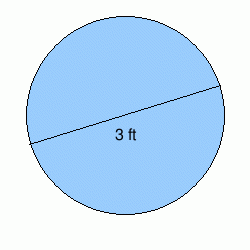Circumference: 2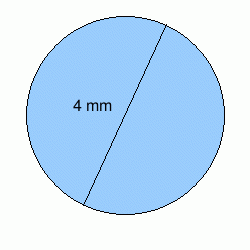Circumference: 3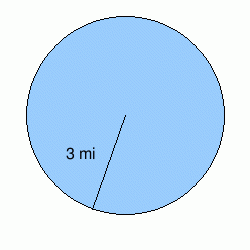Circumference:

Find the circumference. Use 3.14 as an approximation for π.

 1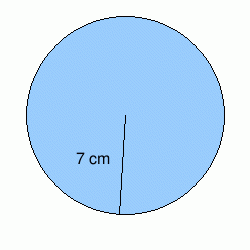Circumference: 2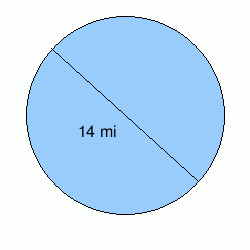Circumference: 3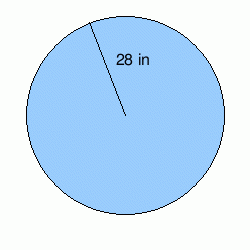Circumference: 4Circumference: 5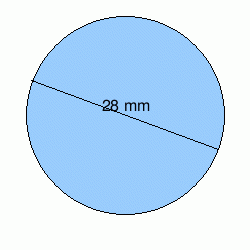Circumference:

Find the circumference. Use 3.14 as an approximation for π.

 1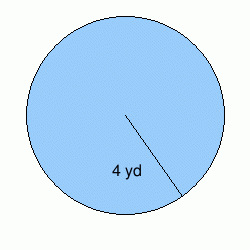Circumference: 2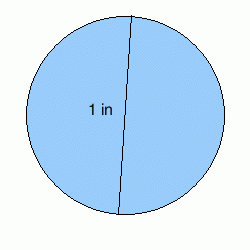Circumference: 3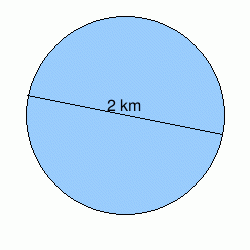Circumference: